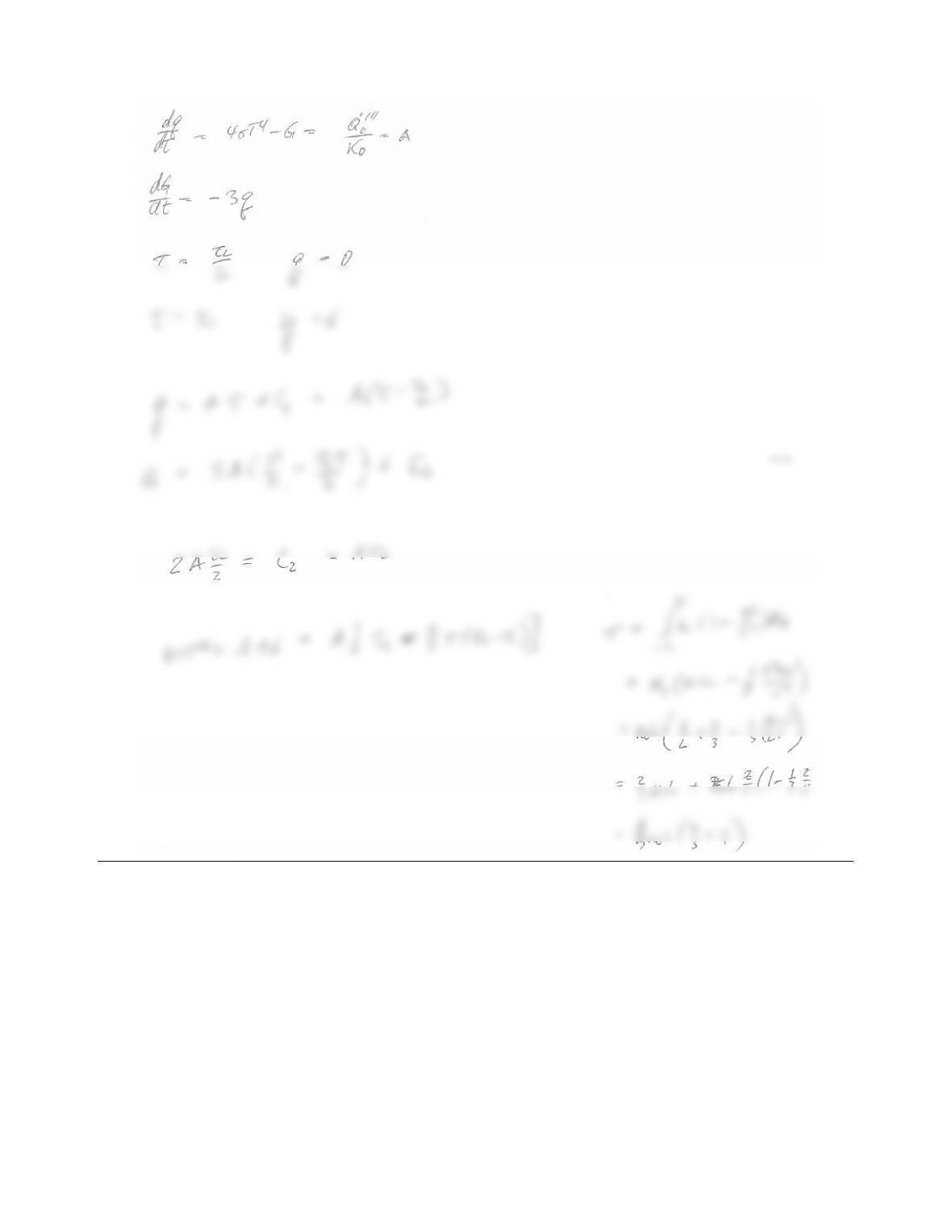# 978-0123869449 Chapter 16 Part 1

Document Type
Homework Help
Book Title
Authors
Michael F. Modest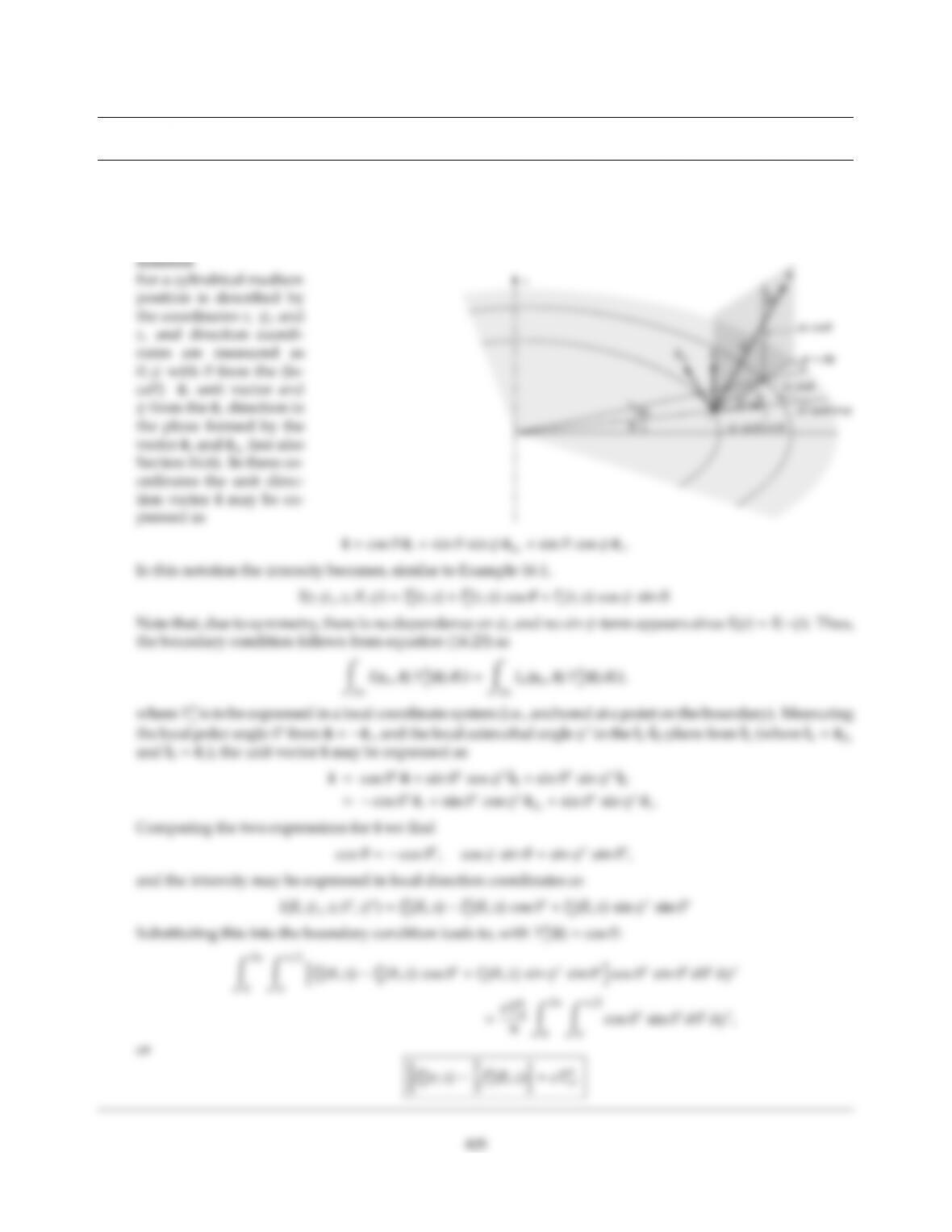CHAPTER 16
16.1 Consider a gray medium at radiative equilibrium contained within a long black cylinder with a surface
temperature of T(r=R,z)=Tw(z). Find the relevant boundary conditions for the P1-approximation directly
from equation (16.23), i.e., in a manner similar to the development in Example 16.1.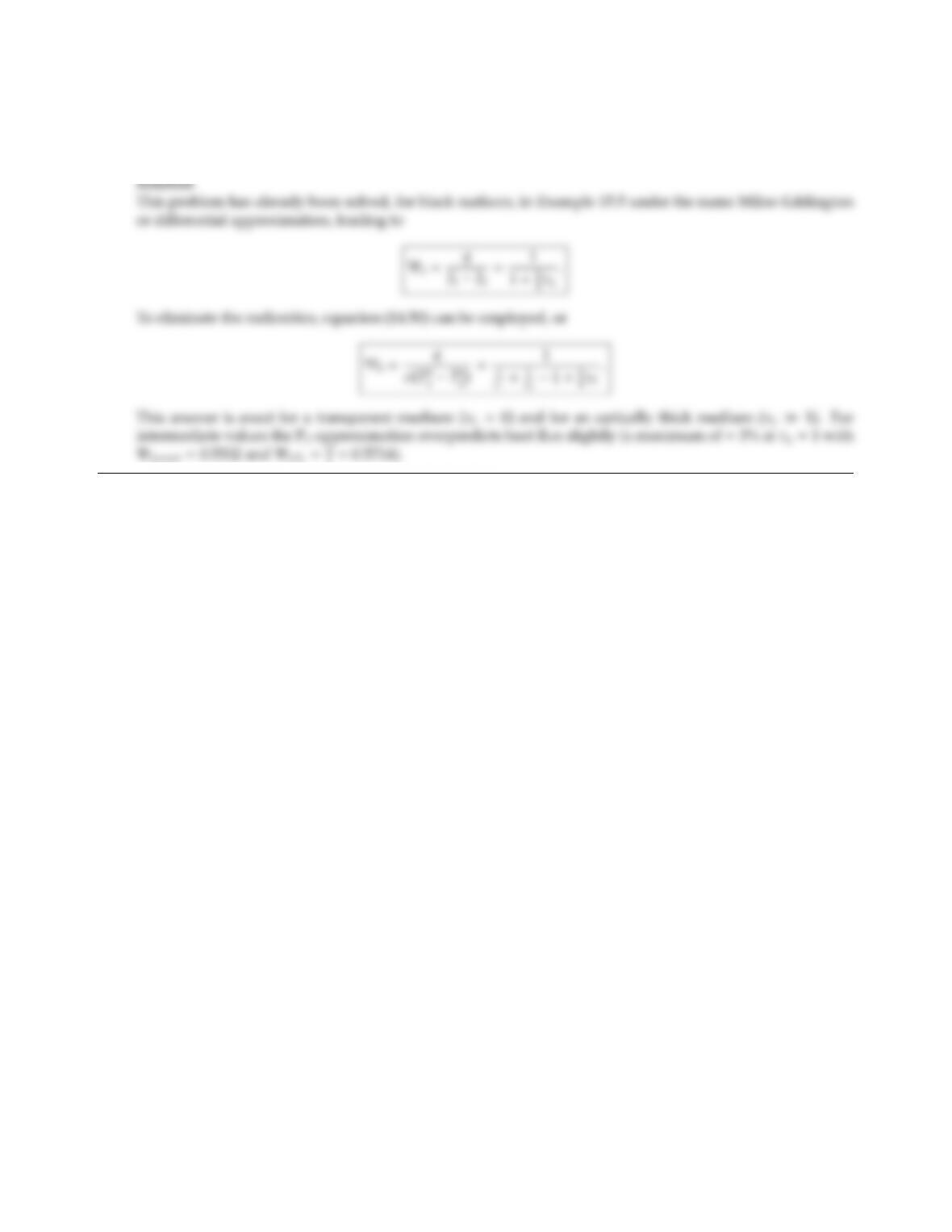16.2 Consider a gray, isotropically scattering medium at radiative equilibrium contained between large, isothermal,
gray plates at temperatures T1and T2, and emittances ǫ1and ǫ2, respectively. Determine the radiative heat ﬂux
between the plates using the P1-approximation. Compare the result with the exact answer from Table 14.1.
7=0.5714).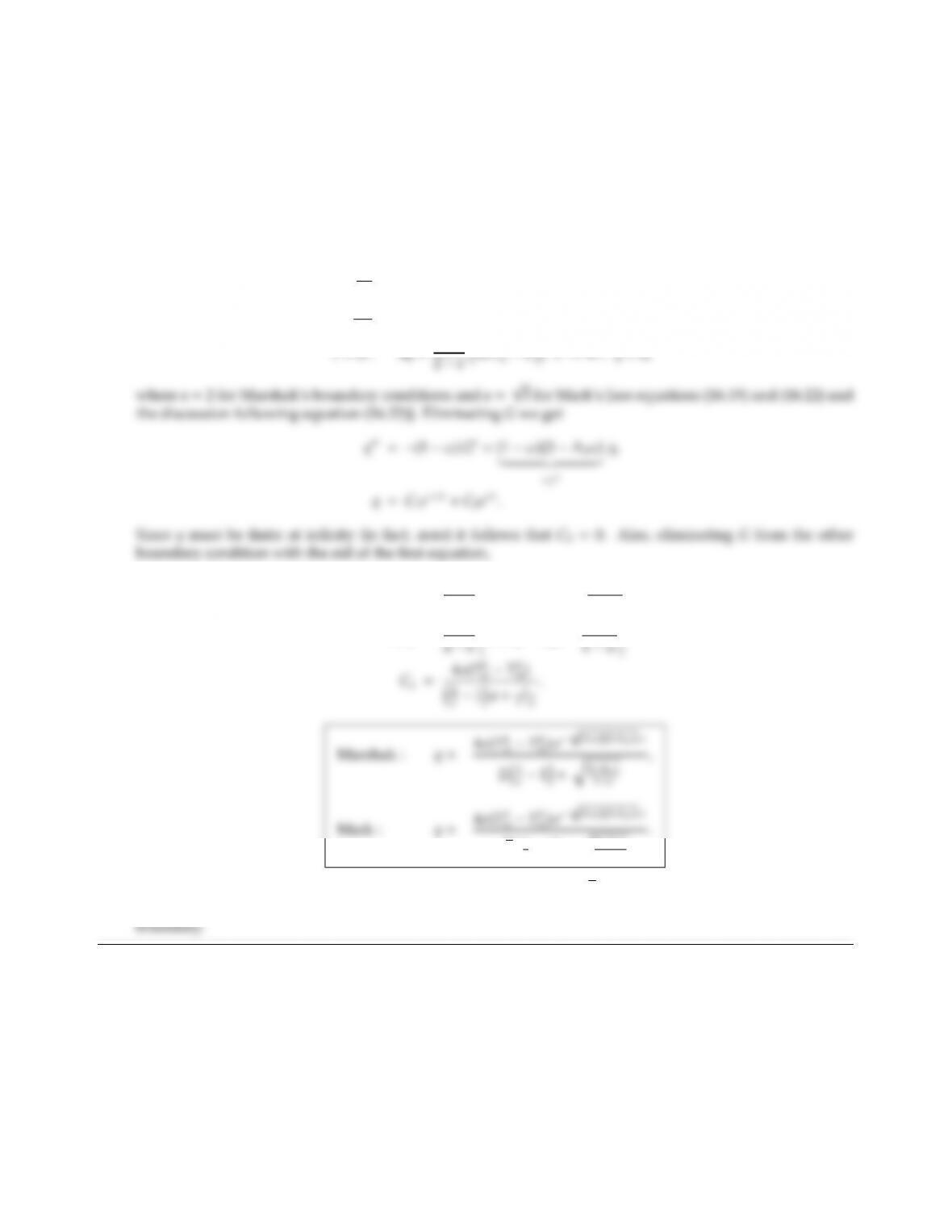CHAPTER 16 407
16.3 Consider a large, isothermal (temperature Tw), gray and diuse (emittance ǫ) wall adjacent to a semi-infinite
gray absorbing/emitting and linear-anisotropically scattering medium. The medium is isothermal (tem-
perature Tm). Determine the radiative heat ﬂux as a function of distance away from the plate using the
P1-approximation with (i) Marshak’s, (ii) Mark’s boundary conditions.
Solution
For a one-dimensional Cartesian coordinate system the P1-approximation is, from equations (16.35), (16.37)
and (16.47),
dq
dτ=(1 ω)(4σT4
mG),
dG
dτ=(3 A1ω)q,
τ=0 : aq =ǫ
2ǫ4σT4
w4σT4
m+1
1ωq
aC1=ǫ
wT4
m)γC1
32
ǫ1+q3A1ω
1ω
For ǫ=1 and ω=0 the heat ﬂux goes to q(T4
wT4
m)=4/a+3=1.0718 (Marshak) or 1.1547 (Mark)
as compared to 1.000 for the exact solution. The error is caused by the temperature discontinuity at the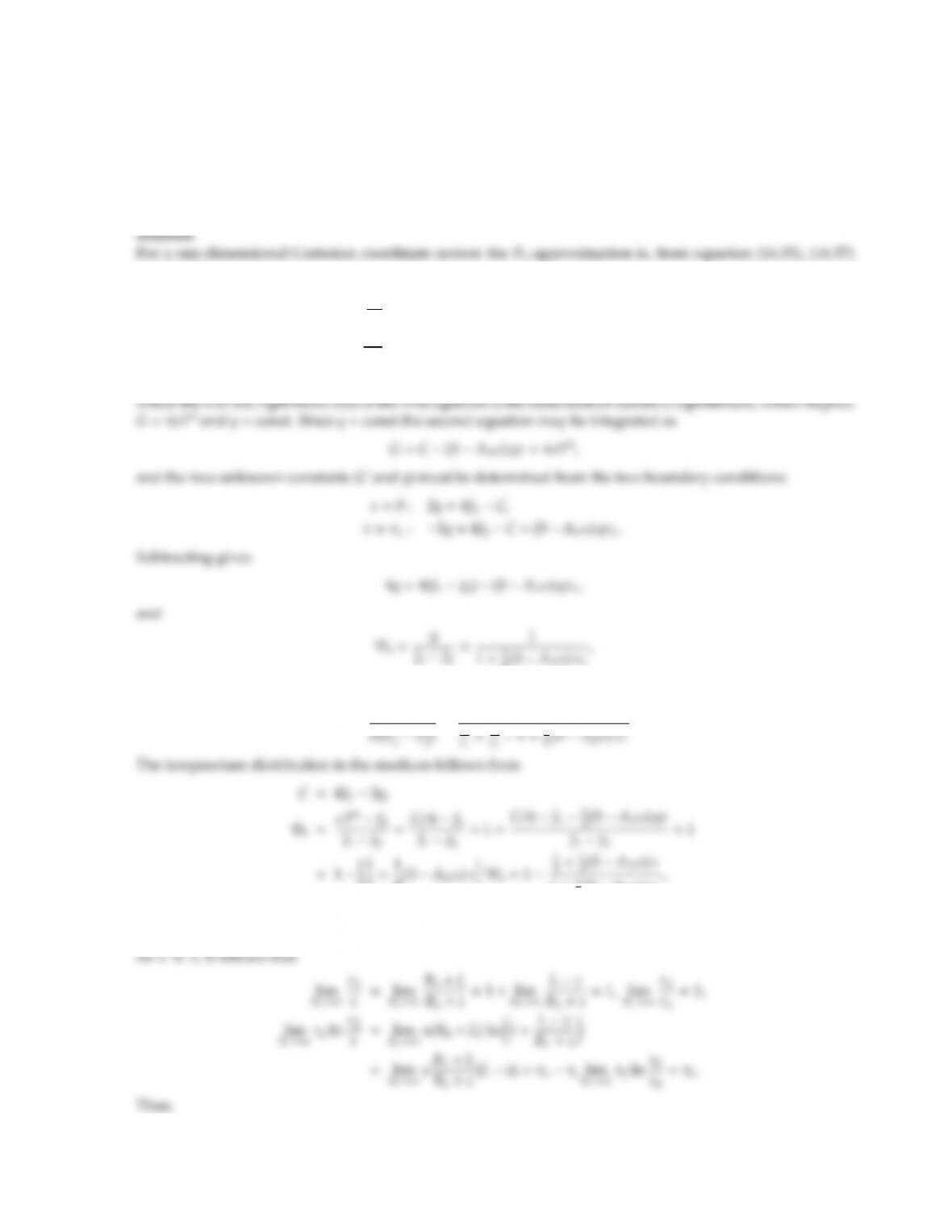16.4 Consider parallel, gray-diuse plates, that are isothermal at temperatures T1and T2, and with emittances ǫ1
and ǫ2, respectively. The plates are separated by a gray, linear-anisotropically scattering medium of thickness
L, which is at radiative equilibrium. Using the P1-approximation, determine the temperature distribution
within, and the heat ﬂux through, the medium. Compare the heat ﬂux with the exact answer given by
Table 14.1 (for isotropic scattering, and optical thicknesses of τL=βL=0,0.1,0.5,1, 2, and 5). Show that the
radiative heat ﬂux can be obtained from the expression given in Example 16.3, by letting R2=R1+L→ ∞.
and (16.47)
dq
dτ=(1 ω)(4σT4
mG)=0,
dG
dτ=(3 A1ω)q,
τ=0 : 2q=4J1G, τ =τL:2q=4J2G,
4(3 A1ω)τL
and with equation (14.50)
Ψ = q
1
.
2+1
4(3 A1ω)τΨb=1
1+1
4(3 A1ω)τL
which reduces to the result of Example 15.5 for A1ω=0.
A similar result is obtained from Example 16.3 by letting R2=R1+L→ ∞. Then, with ln(1 +x)=x+O(x2)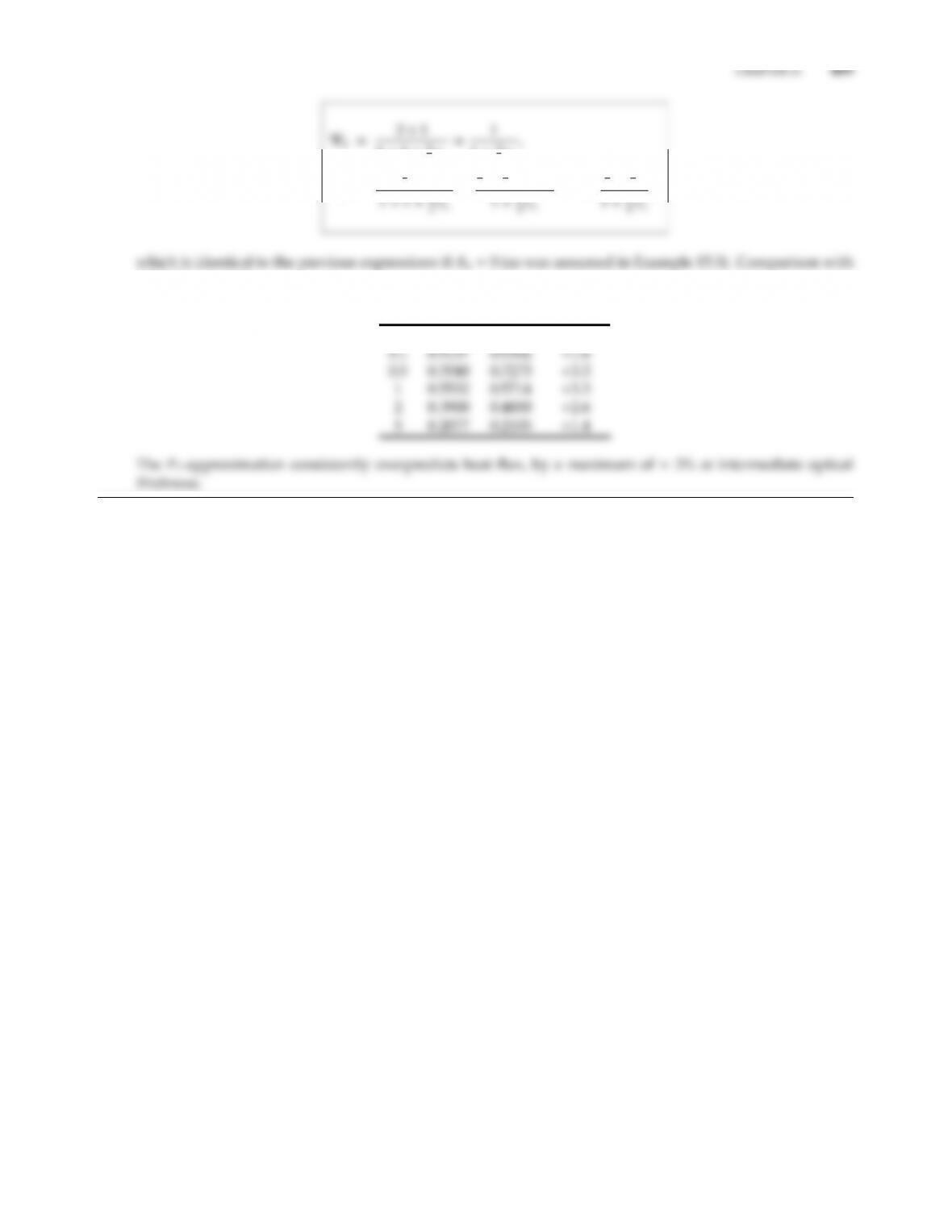1+1+3
2τL
1+3
4τL
Φb=1+3
2(τLτ)
=
1
2+3
4(τLτ)
=1
1
2+3
4τ
,
Table 14.1 reveals:
τLΨb,exact Ψb,P1Error %
0 1 1 0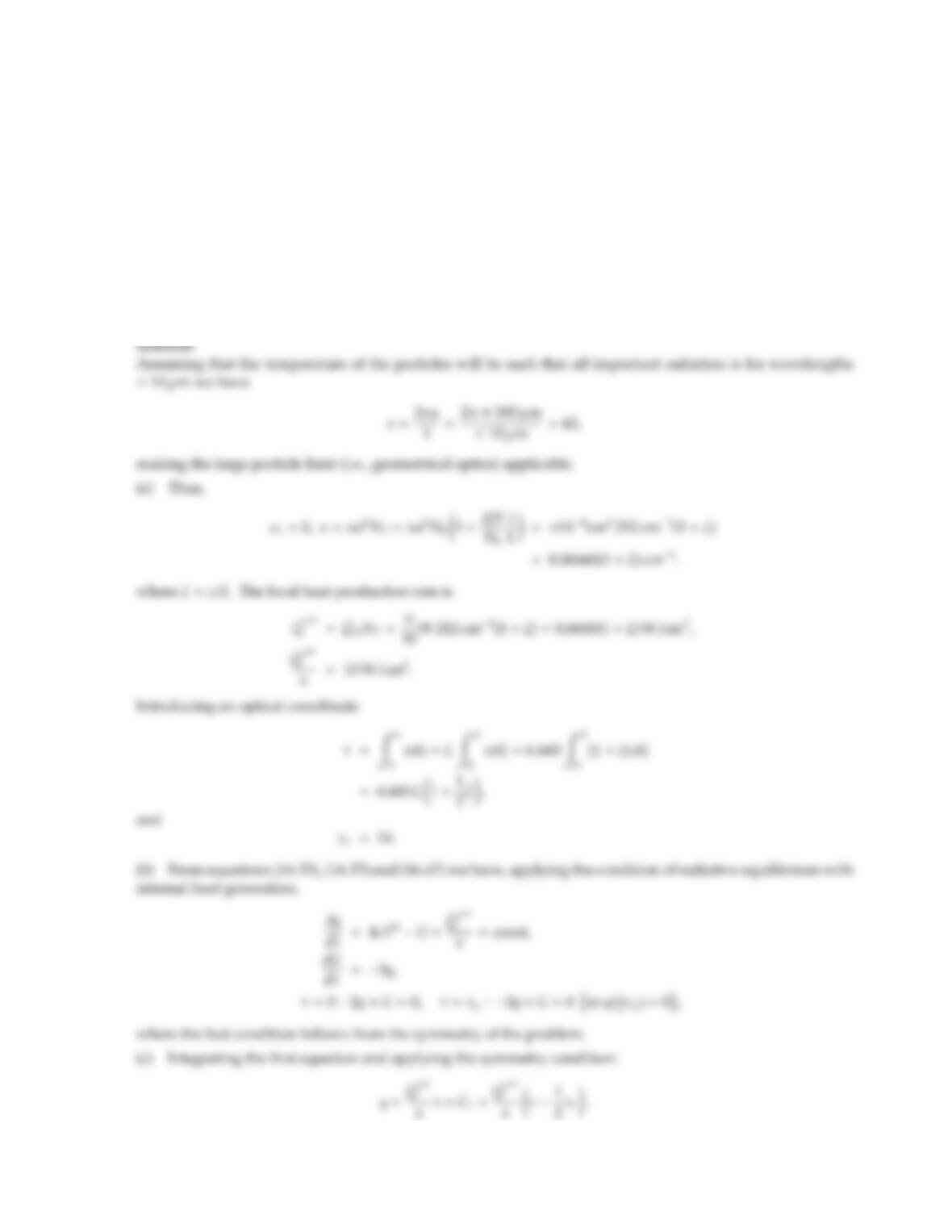16.5 Black spherical particles of 100 µm radius are suspended between two cold and black parallel plates 1 m apart.
The particles produce heat at a rate of π/10 W/particle, which must be removed by thermal radiation. The
number of particles between the plates is given by
NT(z)=N0+ ∆Nz/L,0<z<L;N0= ∆N=212 particles/cm3.
(a) Determine the local absorption coecient and the local heat production rate; introduce an optical
coordinate and determine the optical thickness of the entire gap.
(b) Assuming the P1-approximation to be valid, what are the relevant equations and boundary conditions
governing the heat transfer?
(c) What are the heat ﬂux rates at the top and bottom surfaces? What is the entire amount of energy released
by the particles? What is the maximum particle temperature?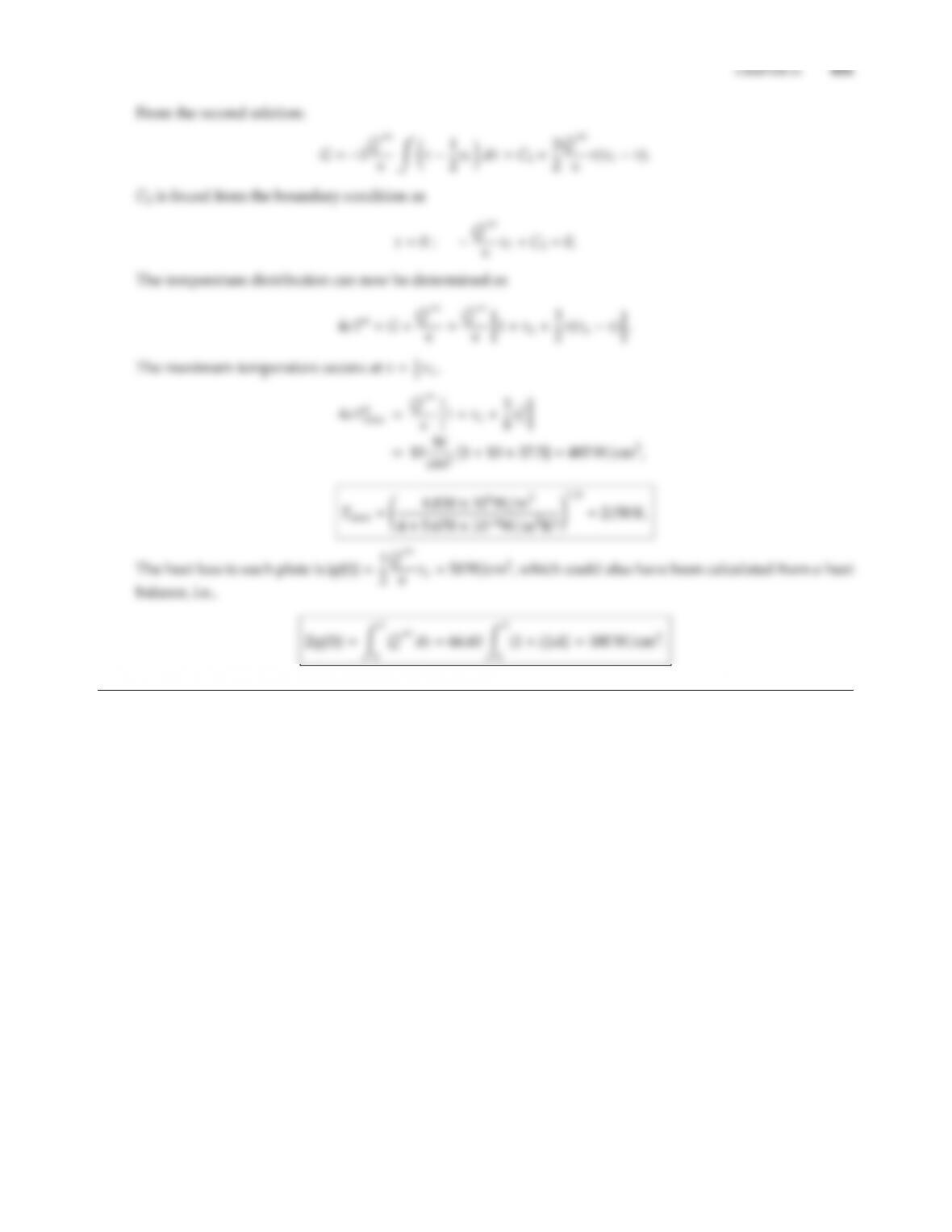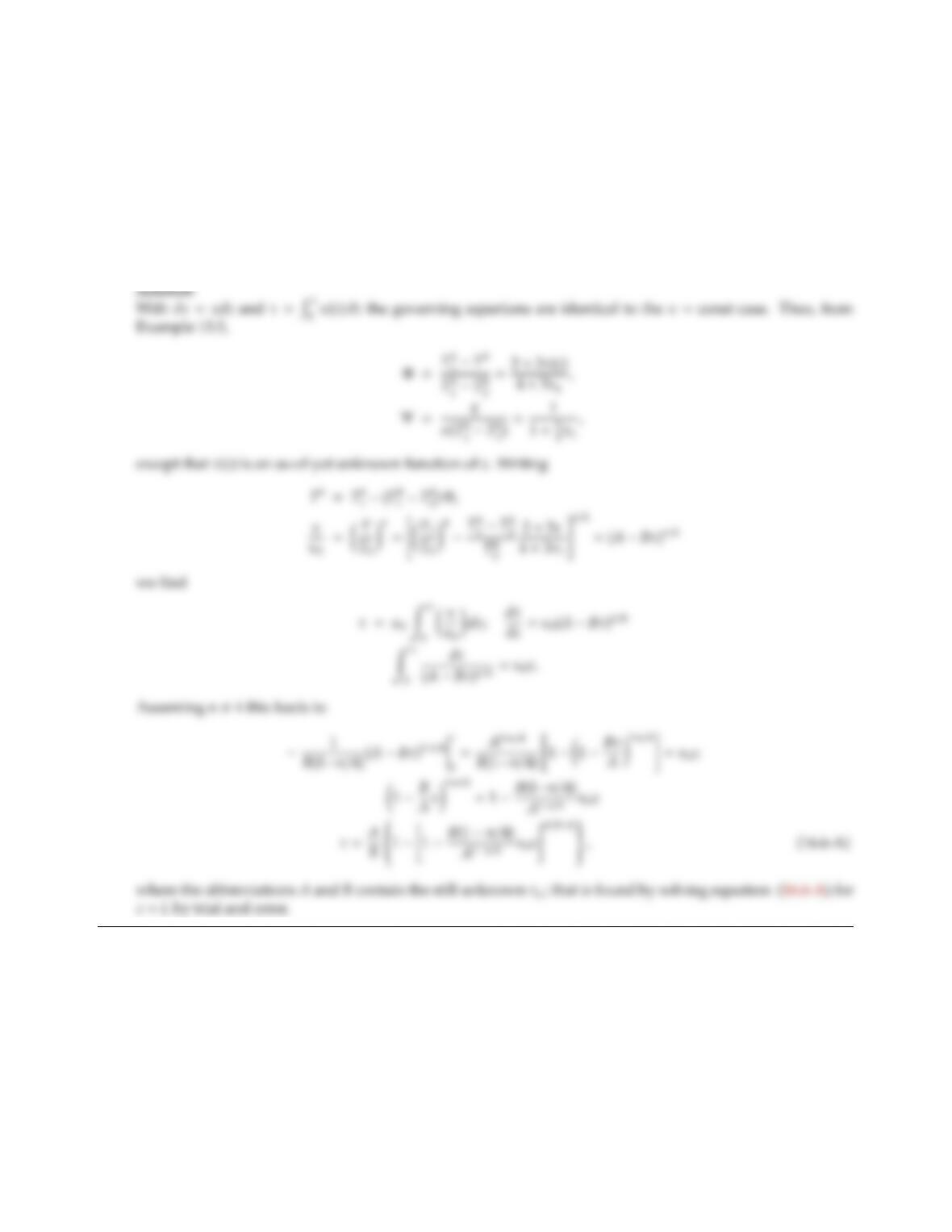16.6 Consider parallel, black plates spaced 1 m apart, at constant temperatures T1=1000 K and T2=300 K,
respectively, separated by a gray, nonscattering medium at radiative equilibrium. The absorption coecient
of the medium depends on its temperature according to a power law, κ=κ0(T/T0)n(κ0=1 m1,T0=300 K,
and nis an arbitrary, positive constant).
(a) Using the P1-approximation, outline how to determine the radiative heat ﬂux through, and temperature
field within, the medium (i.e., the result may contain unsolved implicit relationships).
(b) Write a small computer program to quantify the results for n=0,0.5,1, and 4. Compare with results
obtained for a constant κ[evaluated at an average temperature Tav =0.5×(300+1000) =650 K].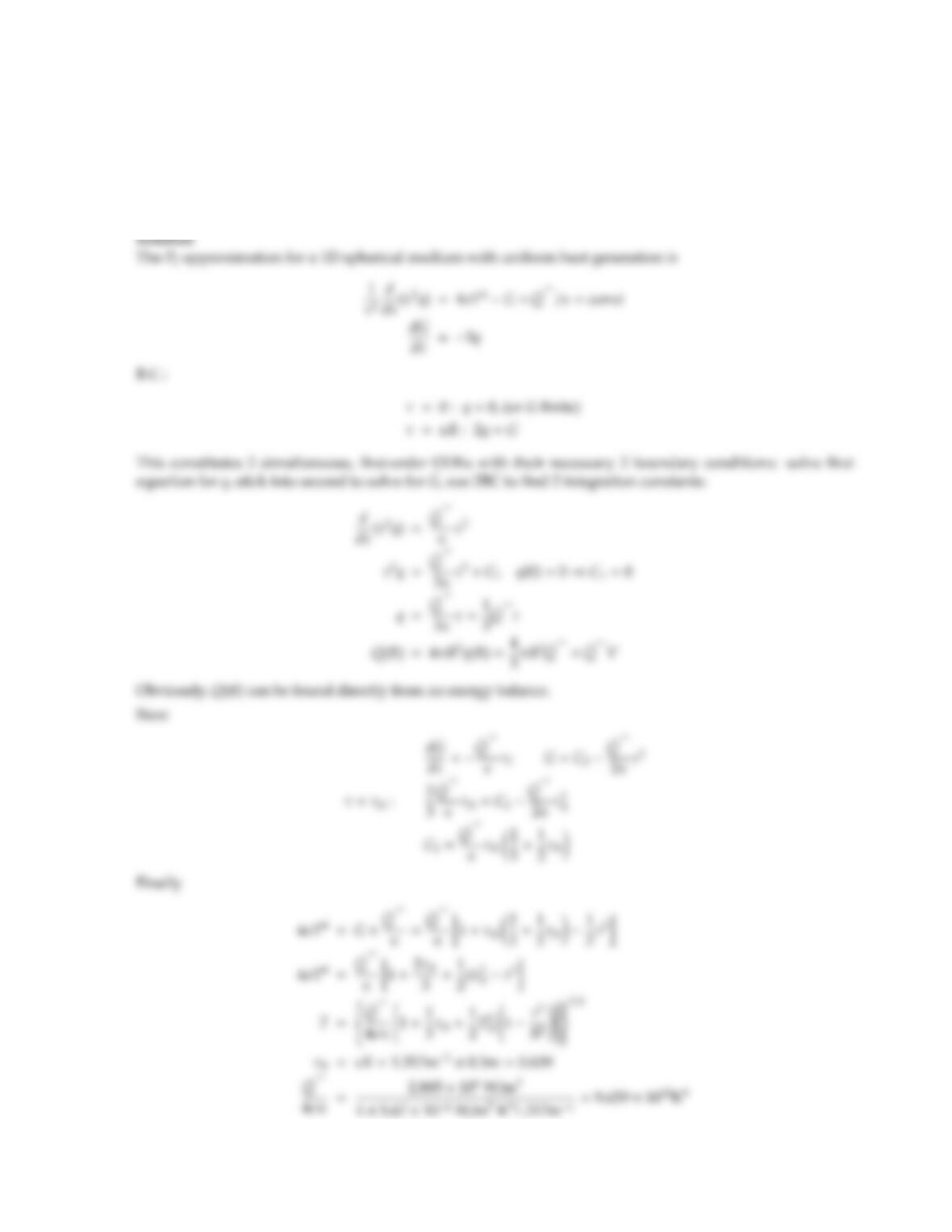CHAPTER 16 413
16.7 A gas/soot/coal mixture, which may be approximated as a gray, nonscattering medium with κ=1.317 m1, is
burning inside a spherical container of diameter D=1 m, which has black and cold walls. During combustion
the coal particles release heat uniformly at a rate of ˙
Q′′′ =2.885 MW/m3(per total volume of container). The
mixture remains at radiative equilibrium throughout the combustion process. Set up the necessary equations
and boundary conditions to find the medium’s temperature distribution and total heat loss rate, assuming
the P1-approximation to hold.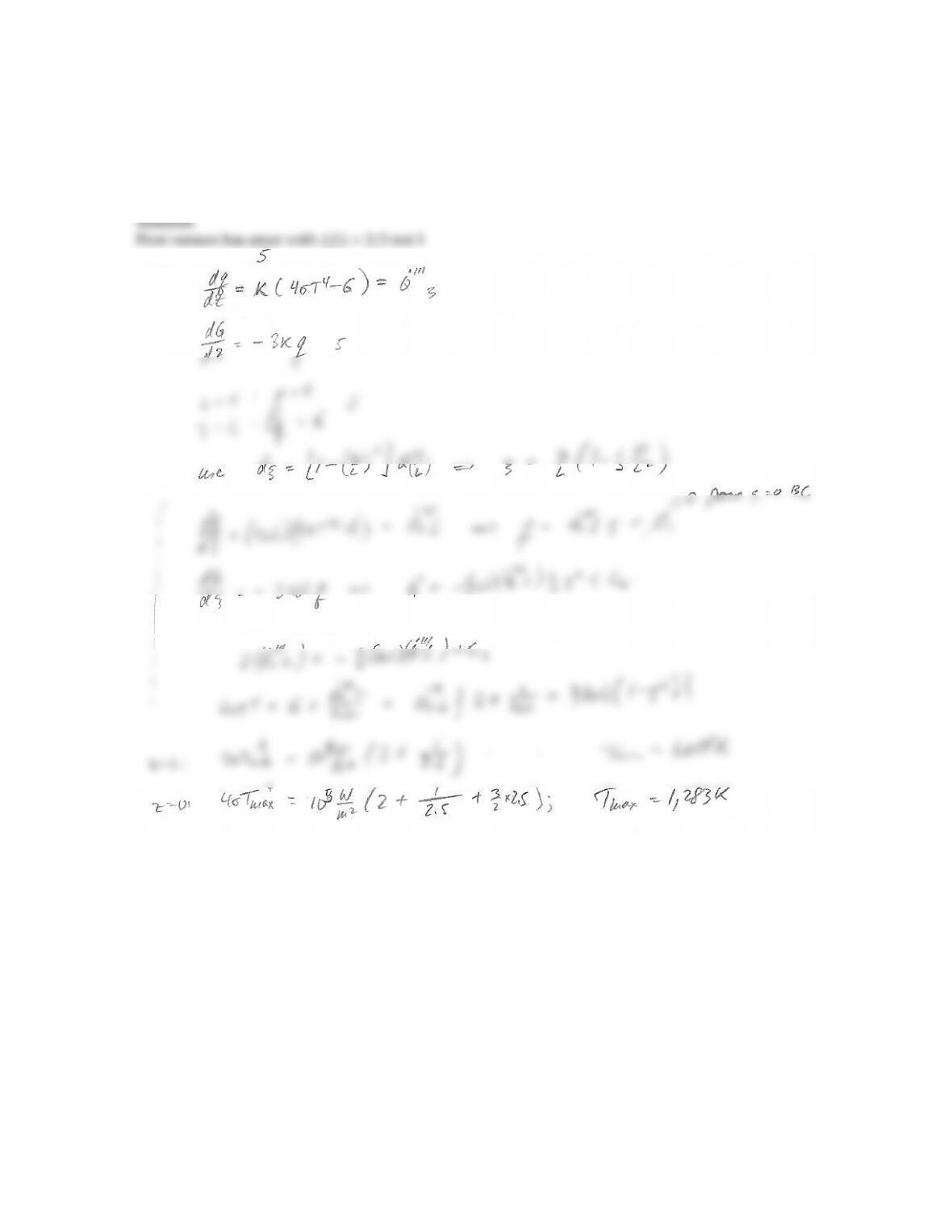CHAPTER 16 415
16.8 Given the soot distribution of Problem 12.16, it is found that soot is generated where combustion takes
place, i.e., the local heat release due to combustion may be written as ˙
Q′′′(z)=˙
Q′′′
0[1 (z/L)2], ˙
Q′′′
0=
105W/m3. Assuming the soot to be nonscattering and gray (with properties evaluated at λ=3µm) and
conduction/convection to be negligible, and the walls to be cold and black, determine the temperature
distribution within the medium using the P1/dierential approximation.